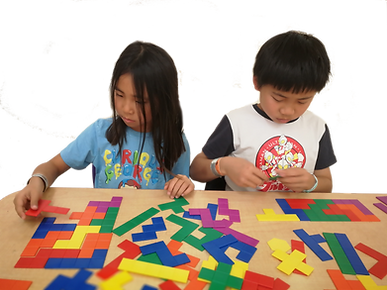top of page

​Mathematical Olympiads are competitive events where participants sit a mathematics test. The test's problems are designed to challenge students' math skills in a variety of areas including analytical skill, creative and imaginative thinking, problem-solving and reasoning.

Our Math Olympiad program introduces different types and topics of problems that students encounter in competitive mathematics. Students are trained to use strategies of problem-solving and to foster mathematical creativity. We develop problem-solving approaches that are not typically covered in school through the language of logic.

We recommend our students to take mathematical contests once they are confident of taking the challenge.What do we do during the class?

• Mental arithmetic training & Number Operations booster

• Math foundation building & Number Sense skills

• Math Olympiad Topic by Topic Demonstration

• Space Imagination & Spatial Sense practice

• Mock test problems practice and prep.

• Individual Q & A

When is the best age to learn?

1st Grade. It depends on each child's math aptitude and interest. Normally, when students are able to read and comprehend math problems, acquire basic mental arithmetic and operations skills, and express great interest in taking challenge problems, they are ready for the Math Olympiad training.

What are the benefits?

• Analytical skill and logical thinking

• Creativity, innovation, and imaginative thinking

• Outside-the-box thinking

• Problem-solving skill

• Excellent mental processing

• Understand how mathematics applies to nature’s laws and human activities.

• Develop a positive attitude towards math

• Self-confidence and self-esteem

• Improve academic performance in schoolHow is Math Olympiad different from the Math covered at school?

The content of Math Olympiad ranges from addition problems that are extremely difficult to problems on branches of mathematics not conventionally covered at school. Often, the problems look complex and difficult but they do not require extensive knowledge of theorem.

Calculus, though allowed in solutions, is never required, as there is a principle at play that anyone with a basic understanding of mathematics should understand the problems, even if the solutions require a great deal more knowledge.

For example, this kind of problem does not require any algebra knowledge and there is a principle at play that even a Grade 2 student can solve it. We will teach students to ungroup and regroup elements, equivalent substitution, solving by comparing and replacement.

Meanwhile, the math covered at school focuses on basic math foundations and repeated practice. The key to success in school math is rote learning and intensive practice on a particular problem. The numbers can look big but it doesn’t require critical and logical skills to solve. On the other hand, Math Olympiad problems require critical thinking and analytical skills to solve. Students have to use strategies to solve these kinds of problems.

Math Olympiad also helps our students understand how mathematics applies to nature’s laws and human activities.

It shows that mathematical education is significant in every part of the world. Our students will be motivated to understand mathematics related to real-life through the use of interesting problems. For example, learning how to optimize the productivity of a certain item and the efficiency of a certain activity. We are aiming to inspire our students for creativity, innovation, and imaginative thinking.

Another highlight of Math Olympiad is that we enrich students’ creativity and imaginative thinking through visual math. Many people have a misunderstanding that math is only related to numbers. In real-life applications, math is significantly related to visual-spatial sense and mental imagination. We provide visual math practice and materials with fun hands-on activities. Students are more likely to be engaged in math-related activities and they will find that math is significant in every part of the world.

​​###### ​

A book costs \$4. A bag costs \$6. Nicholas bought 5 books and 10 bags. How much did he pay in total?

###### ​

A book costs \$4. A bag costs \$6. Nicholas paid \$80 for 15 such books and bags. How many books did he buy?

###### ​

It takes Elise 3 minutes to make a cupcake. How long does it take her to make 12 cupcakes?

###### ​

One frying pan can cook 2 pancakes at a time. A pancake has two sides, each side needs 3 minutes to cook. Assume you have one frying pan, at least how many minutes does it take to cook 3 pancakes?###### ​

Place the items in the cells so that each horizontal line and each vertical line has different kinds of shapes and different kinds of colors.• Math Pioneer Cup Contest

• Vast Ocean Cup Math Contest

• The International Mathematical Olympiad (IMO)

• Math Olympiads for Elementary and Middle Schools (MOEMS)

• Math League

• Math Kangaroo Competition

• American Mathematics Competition (AMC)

• and more!

bottom of page